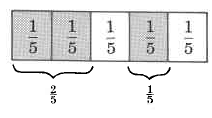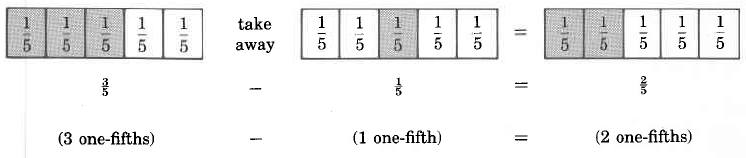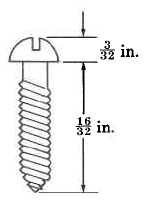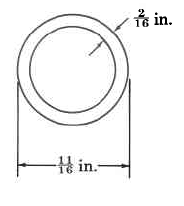# 5.1 Addition and subtraction of fractions with like denominators

 Page 1 / 1
This module is from Fundamentals of Mathematics by Denny Burzynski and Wade Ellis, Jr. This module discusses how to add and subtract fractions with like denominators. By the end of the module students should be able to add and subtract fractions with like denominators.

## Section overview

• Addition of Fraction With Like Denominators
• Subtraction of Fractions With Like Denominators

## Addition of fraction with like denominators

Let's examine the following diagram.2 one-fifths and 1 one fifth is shaded.

It is shown in the shaded regions of the diagram that

(2 one-fifths) + (1 one-fifth) = (3 one-fifths)

That is,

$\frac{2}{5}+\frac{1}{5}=\frac{3}{5}$

From this observation, we can suggest the following rule.

## Method of adding fractions having like denominators

To add two or more fractions that have the same denominators, add the numer­ators and place the resulting sum over the common denominator. Reduce, if necessary.

## Sample set a

Find the following sums.

$\frac{3}{7}+\frac{2}{7}$ . The denominators are the same. Add the numerators and place that sum over 7.

$\frac{3}{7}+\frac{2}{7}=\frac{3+2}{7}=\frac{5}{7}$

$\frac{1}{8}+\frac{3}{8}$ . The denominators are the same. Add the numerators and place the sum over 8. Reduce.

$\frac{1}{8}+\frac{3}{8}=\frac{1+3}{8}=\frac{4}{8}=\frac{1}{2}$

$\frac{4}{9}+\frac{5}{9}$ . The denominators are the same. Add the numerators and place the sum over 9.

$\frac{4}{9}+\frac{5}{9}=\frac{4+5}{9}=\frac{9}{9}=1$

$\frac{7}{8}+\frac{5}{8}$ . The denominators are the same. Add the numerators and place the sum over 8.

$\frac{7}{8}+\frac{5}{8}=\frac{7+5}{8}=\frac{\text{12}}{8}=\frac{3}{2}$

To see what happens if we mistakenly add the denominators as well as the numerators, let's add

$\frac{1}{2}+\frac{1}{2}$

$\frac{1}{2}+\frac{1}{2}=\frac{1+1}{2+2}=\frac{2}{4}=\frac{1}{2}$

This means that two $\frac{1}{2}$ 's is the same as one $\frac{1}{2}$ . Preposterous! We do not add denominators .

## Practice set a

Find the following sums.

$\frac{1}{\text{10}}+\frac{3}{\text{10}}$

$\frac{2}{5}$

$\frac{1}{4}+\frac{1}{4}$

$\frac{1}{2}$

$\frac{7}{\text{11}}+\frac{4}{\text{11}}$

1

$\frac{3}{5}+\frac{1}{5}$

$\frac{4}{5}$

Show why adding both the numerators and denominators is preposterous by adding $\frac{3}{4}$ and $\frac{3}{4}$ and examining the result.

$\frac{3}{4}+\frac{3}{4}=\frac{3+3}{4+4}=\frac{6}{8}=\frac{3}{4}$ , so two $\frac{3}{4}$ ’s= one $\frac{3}{4}$ which is preposterous.

## Subtraction of fractions with like denominators

We can picture the concept of subtraction of fractions in much the same way we pictured addition.From this observation, we can suggest the following rule for subtracting fractions having like denominators:

## Subtraction of fractions with like denominators

To subtract two fractions that have like denominators, subtract the numerators and place the resulting difference over the common denominator. Reduce, if possible.

## Sample set b

Find the following differences.

$\frac{3}{5}-\frac{1}{5}$ . The denominators are the same. Subtract the numerators. Place the difference over 5.

$\frac{3}{5}-\frac{1}{5}=\frac{3-1}{5}=\frac{2}{5}$

$\frac{8}{6}-\frac{2}{6}$ . The denominators are the same. Subtract the numerators. Place the difference over 6.

$\frac{8}{6}-\frac{2}{6}=\frac{8-2}{6}=\frac{6}{6}=1$

$\frac{\text{16}}{9}-\frac{2}{9}$ . The denominators are the same. Subtract numerators and place the difference over 9.

$\frac{\text{16}}{9}-\frac{2}{9}=\frac{\text{16}-2}{9}=\frac{\text{14}}{9}$

To see what happens if we mistakenly subtract the denominators, let's consider

$\frac{7}{\text{15}}-\frac{4}{\text{15}}=\frac{7-4}{\text{15}-\text{15}}=\frac{3}{0}$

We get division by zero, which is undefined. We do not subtract denominators.

## Practice set b

Find the following differences.

$\frac{\text{10}}{\text{13}}-\frac{8}{\text{13}}$

$\frac{2}{\text{13}}$

$\frac{5}{\text{12}}-\frac{1}{\text{12}}$

$\frac{1}{3}$

$\frac{1}{2}-\frac{1}{2}$

0

$\frac{\text{26}}{\text{10}}-\frac{\text{14}}{\text{10}}$

$\frac{6}{5}$

Show why subtracting both the numerators and the denominators is in error by performing the subtraction $\frac{5}{9}-\frac{2}{9}$ .

$\frac{5}{9}-\frac{2}{9}=\frac{5-2}{9-9}=\frac{3}{0}$ , which is undefined

## Exercises

For the following problems, find the sums and differences. Be sure to reduce.

$\frac{3}{8}+\frac{2}{8}$

$\frac{5}{8}$

$\frac{1}{6}+\frac{2}{6}$

$\frac{9}{\text{10}}+\frac{1}{\text{10}}$

1

$\frac{3}{\text{11}}+\frac{4}{\text{11}}$

$\frac{9}{\text{15}}+\frac{4}{\text{15}}$

$\frac{\text{13}}{\text{15}}$

$\frac{3}{\text{10}}+\frac{2}{\text{10}}$

$\frac{5}{\text{12}}+\frac{7}{\text{12}}$

1

$\frac{\text{11}}{\text{16}}-\frac{2}{\text{16}}$

$\frac{3}{\text{16}}-\frac{3}{\text{16}}$

0

$\frac{\text{15}}{\text{23}}-\frac{2}{\text{23}}$

$\frac{1}{6}-\frac{1}{6}$

0

$\frac{1}{4}+\frac{1}{4}+\frac{1}{4}$

$\frac{3}{\text{11}}+\frac{1}{\text{11}}+\frac{5}{\text{11}}$

$\frac{9}{\text{11}}$

$\frac{\text{16}}{\text{20}}+\frac{1}{\text{20}}+\frac{2}{\text{20}}$

$\frac{\text{12}}{8}+\frac{2}{8}+\frac{1}{8}$

$\frac{\text{15}}{8}$

$\frac{1}{\text{15}}+\frac{8}{\text{15}}+\frac{6}{\text{15}}$

$\frac{3}{8}+\frac{2}{\text{8}}-\frac{1}{\text{8}}$

$\frac{1}{2}$

$\frac{\text{11}}{\text{16}}+\frac{9}{\text{16}}-\frac{5}{\text{16}}$

$\frac{4}{\text{20}}-\frac{1}{\text{20}}+\frac{9}{\text{20}}$

$\frac{3}{5}$

$\frac{7}{\text{10}}-\frac{3}{\text{10}}+\frac{\text{11}}{\text{10}}$

$\frac{\text{16}}{5}-\frac{1}{5}-\frac{2}{5}$

$\frac{\text{13}}{5}$

$\frac{\text{21}}{\text{35}}-\frac{\text{17}}{\text{35}}+\frac{\text{31}}{\text{35}}$

$\frac{5}{2}+\frac{\text{16}}{2}-\frac{1}{2}$

10

$\frac{1}{\text{18}}+\frac{3}{\text{18}}+\frac{1}{\text{18}}+\frac{4}{\text{18}}-\frac{5}{\text{18}}$

$\frac{6}{\text{22}}-\frac{2}{\text{22}}+\frac{4}{\text{22}}-\frac{1}{\text{22}}+\frac{\text{11}}{\text{22}}$

$\frac{9}{\text{11}}$

The following rule for addition and subtraction of two fractions is preposterous. Show why by performing the operations using the rule for the following two problems.

## Preposterous rule

To add or subtract two fractions, simply add or subtract the numerators and place this result over the sum or difference of the denominators.

$\frac{3}{\text{10}}-\frac{3}{\text{10}}$

$\frac{8}{\text{15}}+\frac{8}{\text{15}}$

$\frac{\text{16}}{\text{30}}=\frac{8}{5}$ (using the preposterous rule)

Find the total length of the screw.Two months ago, a woman paid off $\frac{3}{\text{24}}$ of a loan. One month ago, she paid off $\frac{5}{\text{24}}$ of the total loan. This month she will again pay off $\frac{5}{\text{24}}$ of the total loan. At the end of the month, how much of her total loan will she have paid off?

$\frac{\text{13}}{\text{24}}$

Find the inside diameter of the pipe.## Exercises for review

( [link] ) Round 2,650 to the nearest hundred.

2700

( [link] ) Use the numbers 2, 4, and 8 to illustrate the associative property of addition.

( [link] ) Find the prime factors of 495.

${3}^{2}\cdot 5\cdot \text{11}$

( [link] ) Find the value of $\frac{3}{4}\cdot \frac{\text{16}}{\text{25}}\cdot \frac{5}{9}$ .

( [link] ) $\frac{8}{3}$ of what number is $1\frac{7}{9}$ ?

$\frac{2}{3}$

where we get a research paper on Nano chemistry....?
nanopartical of organic/inorganic / physical chemistry , pdf / thesis / review
Ali
what are the products of Nano chemistry?
There are lots of products of nano chemistry... Like nano coatings.....carbon fiber.. And lots of others..
learn
Even nanotechnology is pretty much all about chemistry... Its the chemistry on quantum or atomic level
learn
da
no nanotechnology is also a part of physics and maths it requires angle formulas and some pressure regarding concepts
Bhagvanji
hey
Giriraj
Preparation and Applications of Nanomaterial for Drug Delivery
revolt
da
Application of nanotechnology in medicine
what is variations in raman spectra for nanomaterials
ya I also want to know the raman spectra
Bhagvanji
I only see partial conversation and what's the question here!
what about nanotechnology for water purification
please someone correct me if I'm wrong but I think one can use nanoparticles, specially silver nanoparticles for water treatment.
Damian
yes that's correct
Professor
I think
Professor
Nasa has use it in the 60's, copper as water purification in the moon travel.
Alexandre
nanocopper obvius
Alexandre
what is the stm
is there industrial application of fullrenes. What is the method to prepare fullrene on large scale.?
Rafiq
industrial application...? mmm I think on the medical side as drug carrier, but you should go deeper on your research, I may be wrong
Damian
How we are making nano material?
what is a peer
What is meant by 'nano scale'?
What is STMs full form?
LITNING
scanning tunneling microscope
Sahil
how nano science is used for hydrophobicity
Santosh
Do u think that Graphene and Fullrene fiber can be used to make Air Plane body structure the lightest and strongest. Rafiq
Rafiq
what is differents between GO and RGO?
Mahi
what is simplest way to understand the applications of nano robots used to detect the cancer affected cell of human body.? How this robot is carried to required site of body cell.? what will be the carrier material and how can be detected that correct delivery of drug is done Rafiq
Rafiq
if virus is killing to make ARTIFICIAL DNA OF GRAPHENE FOR KILLED THE VIRUS .THIS IS OUR ASSUMPTION
Anam
analytical skills graphene is prepared to kill any type viruses .
Anam
Any one who tell me about Preparation and application of Nanomaterial for drug Delivery
Hafiz
what is Nano technology ?
write examples of Nano molecule?
Bob
The nanotechnology is as new science, to scale nanometric
brayan
nanotechnology is the study, desing, synthesis, manipulation and application of materials and functional systems through control of matter at nanoscale
Damian
Is there any normative that regulates the use of silver nanoparticles?
what king of growth are you checking .?
Renato
What fields keep nano created devices from performing or assimulating ? Magnetic fields ? Are do they assimilate ?
why we need to study biomolecules, molecular biology in nanotechnology?
?
Kyle
yes I'm doing my masters in nanotechnology, we are being studying all these domains as well..
why?
what school?
Kyle
biomolecules are e building blocks of every organics and inorganic materials.
Joe
From 1973 to 1979, in the United States, there was an increase of 166.6% of Ph.D. social scien­tists to 52,000. How many were there in 1973?
7hours 36 min - 4hours 50 minBy OpenStaxBy OpenStaxBy Keyaira BraxtonBy Anonymous UserBy OpenStaxBy Mahee BooBy OpenStaxByBy OpenStaxBy Carly Allen